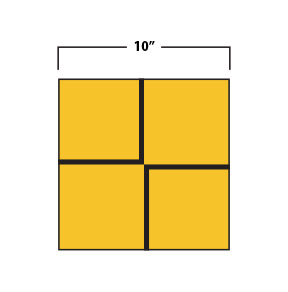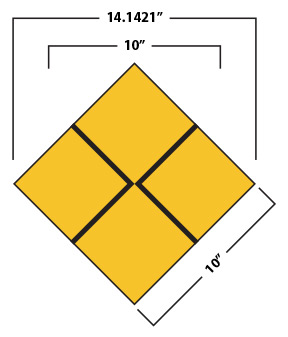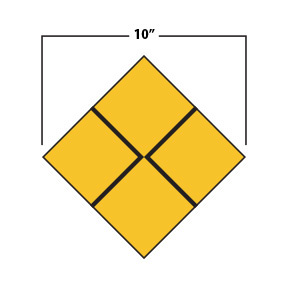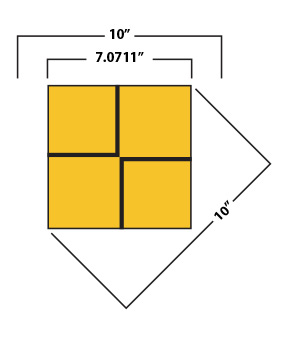### Why It Is Important To Understand Shapes And Sizes.

All shapes are not equally sized. If an item being ordered is square shaped or diamond shaped, it is important to know whether the dimensions provided are for the square size or for the diamond size. In the end, we need to know the overall square size, but in order to determine the correct dimensions for that overall size, we need to know which shape the customer is measuring the dimensions for.

So, let’s take a look at how size matters when you’re talking about shapes that are square or diamond.

#### Square

We’ll begin by looking at a 10” x 10” square.Rotating the square 45° would change its shape to that of a diamond. The diamond’s points would now extend wider than the square’s sides did, therefore changing the overall dimensions as well as the shape.

#### Square Rotated 45°For example, in the picture to the right, there are three measurements:

1. A 10” measurement to show that the side still measures 10”
2. A 10” measurement to show where the square was
3. A 14.1421” measurement to show the new width of the diamond from that square.

#### Diamond

Now let’s take a look at a 10″ diamond.Rotating the diamond 45° would change its shape to that of a square. The square’s sides would no longer be as wide as the diamond’s sides were, again changing the overall dimensions as well as the shape.

#### Diamond Rotated 45°For example, in the picture to the right, there are three measurements:

1. A 10” measurement to show that the points still measures 10”
2. A 10” measurement to show where the diamond was
3. A 7.0711” measurement to show the new width of the square from that diamond.

#### Conclusion

So, from the illustrations above, you can now see why it’s important to know which shape you are measuring for. Just because an art file is sent as a square, doesn’t mean that the measurements aren’t for a diamond. Knowing which shape is being measured will prevent the product from being the wrong size.